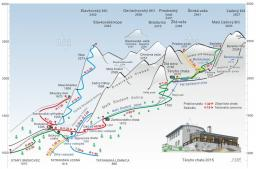# Paul and Honza

Honza went to chat on a bike without a break 4.0 hours. Paul went the same way without breaks 50 minutes longer. The difference between the speeds of the two boys was one kilometer per hour. How fast was Paul if you know that the boys' speed did not change during the journey?

p =  0 km/h

### Step-by-step explanation:

The system of equations is non-linear.

Our linear equations calculator calculates it.Did you find an error or inaccuracy? Feel free to write us. Thank you!

Tips for related online calculators
Do you have a linear equation or system of equations and looking for its solution? Or do you have a quadratic equation?
Do you want to convert length units?
Do you want to convert velocity (speed) units?
Do you want to convert time units like minutes to seconds?